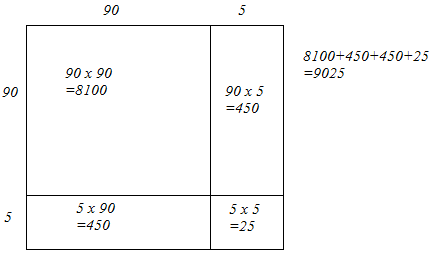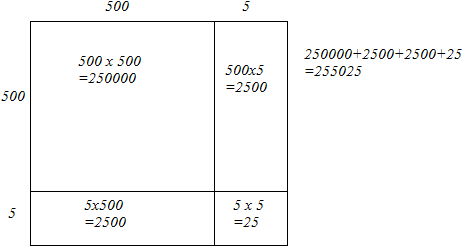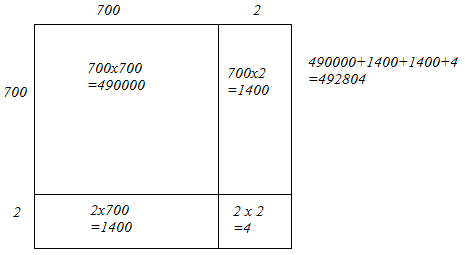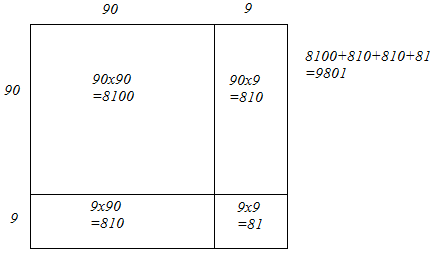# Find the squares of the following numbers by visual method:`
Question:

Find the squares of the following numbers by visual method:

(i) 52

(ii) 95

(iii) 505

(iv) 702

(v) 99

Solution:

(i) We have:

52 = 50 + 2

Let us draw a square having side 52 units. Let us split it into 50 units and 2 units.The sum of the areas of these four parts is the square of 52. Thus, the square of 52 is 2704.

(ii) We have:

95 = 90 + 5

Let us draw a square having side 95 units. Let us split it into 90 units and 5 units.The sum of the areas of these four parts is the square of 95. Thus, the square of 95 is 9025.

(iii) We have:

505 = 500 + 5

Let us draw a square having side 505 units. Let us split it into 500 units and 5 units.The sum of the areas of these four parts is the square of 505. Thus, the square of 505 is 255025.

(iv) We have:

702 = 700 + 2

Let us draw a square having side 702 units. Let us split it into 700 units and 2 units.The sum of the areas of these four parts is the square of 702. Thus, the square of 702 is 492804.

(v) We have:

99 = 90 + 9

Let us draw a square having side 99 units. Let us split it into 90 units and 9 units.The sum of the areas of these four parts is the square of 99. Thus, the square of 99 is 9801.